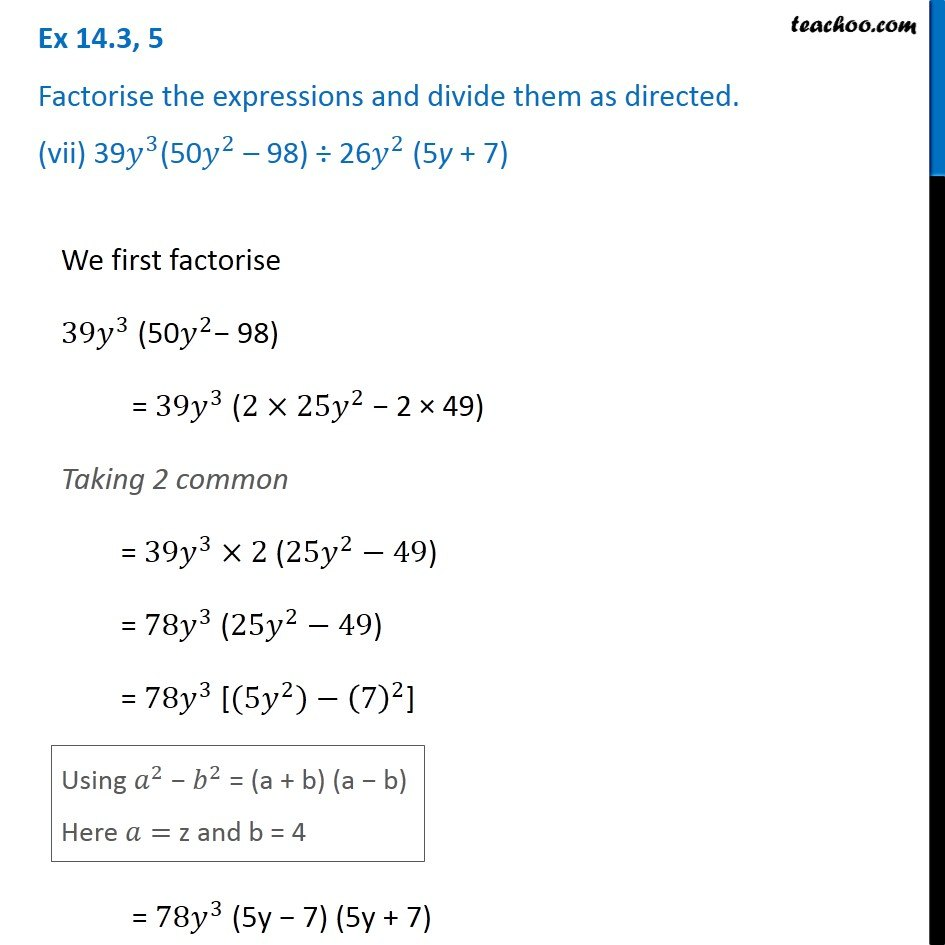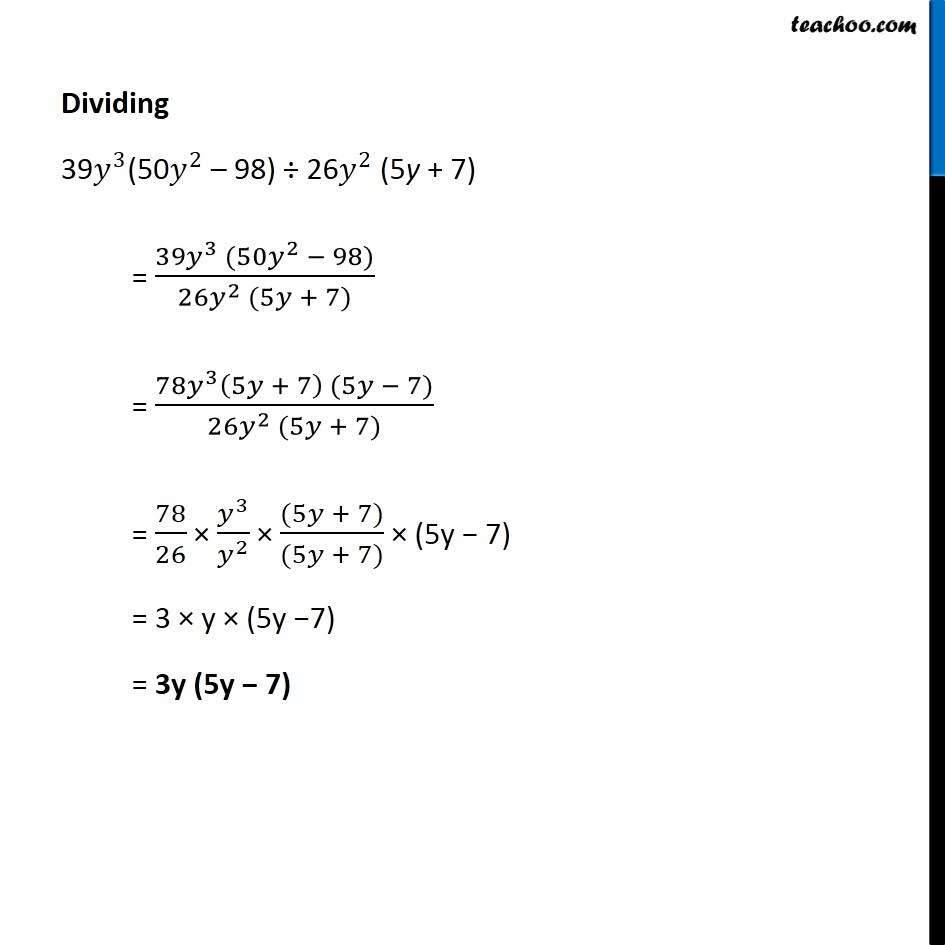Ex 14.3

Chapter 14 Class 8 Factorisation
Serial order wiseGet live Maths 1-on-1 Classs - Class 6 to 12

### Transcript

Ex 14.3, 5 Factorise the expressions and divide them as directed. (vii) 39𝑦^3(50𝑦^2 – 98) ÷ 26𝑦^2 (5y + 7) We first factorise 〖39𝑦〗^3 (50𝑦^2− 98) = 〖39𝑦〗^3 (2×〖25𝑦〗^2 − 2 × 49) Taking 2 common = 〖39𝑦〗^3×2 (〖25𝑦〗^2−49) = 〖78𝑦〗^3 (〖25𝑦〗^2−49) = 〖78𝑦〗^3 [(〖5𝑦〗^2)−(7)^2] Using 𝑎^2 − 𝑏^2 = (a + b) (a − b) Here 𝑎= z and b = 4 Dividing 39𝑦^3(50𝑦^2 – 98) ÷ 26𝑦^2 (5y + 7) = (39𝑦^3 (50𝑦^2 − 98))/(26𝑦^2 (5𝑦 + 7)) = (78𝑦^3 (5𝑦 + 7) (5𝑦 − 7))/(26𝑦^2 (5𝑦 + 7)) = 78/26 × 𝑦^3/𝑦^2 × ((5𝑦 + 7))/((5𝑦 + 7)) × (5y − 7) = 3 × y × (5y −7) = 3y (5y − 7)